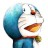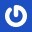# 杰拉斯的博客

## [HDU_ACM_Steps]Chapter One Section One杰拉斯 | 时间：2012-07-23, Mon | 12,655 views

### A+B for Input-Output Practice (I)

Time Limit: 2000/1000 MS (Java/Others) Memory Limit: 65536/32768 K (Java/Others)
Total Submission(s): 13098 Accepted Submission(s): 6187

#### Description

Too easy?! Of course! I specially designed the problem for acm beginners.
You must have found that some problems have the same titles with this one, yes, all these problems were designed for the same aim.

#### Input

The input will consist of a series of pairs of integers a and b, separated by a space, one pair of integers per line.

#### Output

For each pair of input integers a and b you should output the sum of a and b in one line, and with one line of output for each line in input.

```
1 5
10 20
```

#### Sample Output

```6
30#include<stdio.h>
int main(){
int a, b;
while(~scanf("%d%d", &a, &b))
printf("%d\n", a + b);
return 0;
}
A+B for Input-Output Practice (II)Time Limit: 2000/1000 MS (Java/Others) Memory Limit: 65536/32768 K (Java/Others)
Total Submission(s): 7593 Accepted Submission(s): 5398DescriptionYour task is to Calculate a + b.InputInput contains an integer N in the first line, and then N lines follow. Each line consists of a pair of integers a and b, separated by a space, one pair of integers per line.OutputFor each pair of input integers a and b you should output the sum of a and b in one line, and with one line of output for each line in input.Sample Input
2
1 5
10 20
Sample Output6
30#include<stdio.h>
int main(){
int a, b, t;
scanf("%d", &t);
while(t--){
scanf("%d%d",&a, &b);
printf("%d\n", a + b);
}
return 0;
}
A+B for Input-Output Practice (III)Time Limit: 2000/1000 MS (Java/Others) Memory Limit: 65536/32768 K (Java/Others)
Total Submission(s): 8870 Accepted Submission(s): 5142DescriptionYour task is to Calculate a + b.InputInput contains multiple test cases. Each test case contains a pair of integers a and b, one pair of integers per line. A test case containing 0 0 terminates the input and this test case is not to be processed.OutputFor each pair of input integers a and b you should output the sum of a and b in one line, and with one line of output for each line in input.Sample Input
1 5
10 20
0 0
Sample Output6
30#include<stdio.h>
int main(){
int a, b, t;
while(~scanf("%d%d", &a, &b) && a || b)
printf("%d\n", a + b);
return 0;
}
A+B for Input-Output Practice (IV)Time Limit: 2000/1000 MS (Java/Others) Memory Limit: 65536/32768 K (Java/Others)
Total Submission(s): 7942 Accepted Submission(s): 4814DescriptionYour task is to Calculate the sum of some integers.InputInput contains multiple test cases. Each test case contains a integer N, and then N integers follow in the same line. A test case starting with 0 terminates the input and this test case is not to be processed.OutputFor each group of input integers you should output their sum in one line, and with one line of output for each line in input.Sample Input
4 1 2 3 4
5 1 2 3 4 5
0
Sample Output10
15#include<stdio.h>
int main(){
int t, num, sum;
while(~scanf("%d", &t) && t){
sum = 0;
while(t--){
scanf("%d", &num);
sum += num;
}
printf("%d\n", sum);
}
return 0;
}
A+B for Input-Output Practice (V)Time Limit: 2000/1000 MS (Java/Others) Memory Limit: 65536/32768 K (Java/Others)
Total Submission(s): 6184 Accepted Submission(s): 4653DescriptionYour task is to calculate the sum of some integers.InputInput contains an integer N in the first line, and then N lines follow. Each line starts with a integer M, and then M integers follow in the same line.OutputFor each group of input integers you should output their sum in one line, and with one line of output for each line in input.Sample Input
2
4 1 2 3 4
5 1 2 3 4 5
Sample Output10
15#include<stdio.h>
int main(){
int n, t, num, sum;
scanf("%d", &t);
while(t--){
sum = 0;
scanf("%d", &n);
while(n--){
scanf("%d", &num);
sum += num;
}
printf("%d\n", sum);
}
return 0;
}
A+B for Input-Output Practice (VI)Time Limit: 2000/1000 MS (Java/Others) Memory Limit: 65536/32768 K (Java/Others)
Total Submission(s): 6284 Accepted Submission(s): 4538DescriptionYour task is to calculate the sum of some integers.InputInput contains multiple test cases, and one case one line. Each case starts with an integer N, and then N integers follow in the same line.OutputFor each test case you should output the sum of N integers in one line, and with one line of output for each line in input.Sample Input
4 1 2 3 4
5 1 2 3 4 5
Sample Output10
15#include<stdio.h>
int main(){
int n, num, sum;
while(~scanf("%d", &n)){
sum = 0;
while(n--){
scanf("%d", &num);
sum += num;
}
printf("%d\n", sum);
}
return 0;
}
A+B for Input-Output Practice (VII)Time Limit: 2000/1000 MS (Java/Others) Memory Limit: 65536/32768 K (Java/Others)
Total Submission(s): 6634 Accepted Submission(s): 4496DescriptionYour task is to Calculate a + b.InputThe input will consist of a series of pairs of integers a and b, separated by a space, one pair of integers per line.OutputFor each pair of input integers a and b you should output the sum of a and b, and followed by a blank line.Sample Input
1 5
10 20
Sample Output630#include<stdio.h>
int main(){
int a, b;
while(~scanf("%d%d", &a, &b))
printf("%d\n\n", a + b);
return 0;
}
A+B for Input-Output Practice (VIII)Time Limit: 2000/1000 MS (Java/Others) Memory Limit: 65536/32768 K (Java/Others)
Total Submission(s): 13966 Accepted Submission(s): 4508DescriptionYour task is to calculate the sum of some integers.InputInput contains an integer N in the first line, and then N lines follow. Each line starts with a integer M, and then M integers follow in the same line.OutputFor each group of input integers you should output their sum in one line, and you must note that there is a blank line between outputs.Sample Input
3
4 1 2 3 4
5 1 2 3 4 5
3 1 2 3
Sample Output10156#include<stdio.h>
int main(){
int t, n, num, sum;
scanf("%d", &t);
while(t--){
sum = 0;
scanf("%d", &n);
while(n--){
scanf("%d", &num);
sum += num;
}
printf("%d\n", sum);
if(t > 0){
printf("\n");
}
}
return 0;
}

相关文章

[ACM_Tyvj_1011]传纸条（双线程动态规划解法）
[ACM_NYOJ_19]擅长排列的小明
[ACM_NYOJ_47]过河问题
[ACM_NYOJ_27]水池数目
[ACM_NYOJ_8]一种排序

```

#### 2 条评论 »

1.蜗牛都知道

Test Comment.

1.蓝飞

明显是可以的= =。。